# Bayesian statistics overview

hahajjjun·2022년 12월 4일
0

## Bayesian Statistics

목록 보기
1/2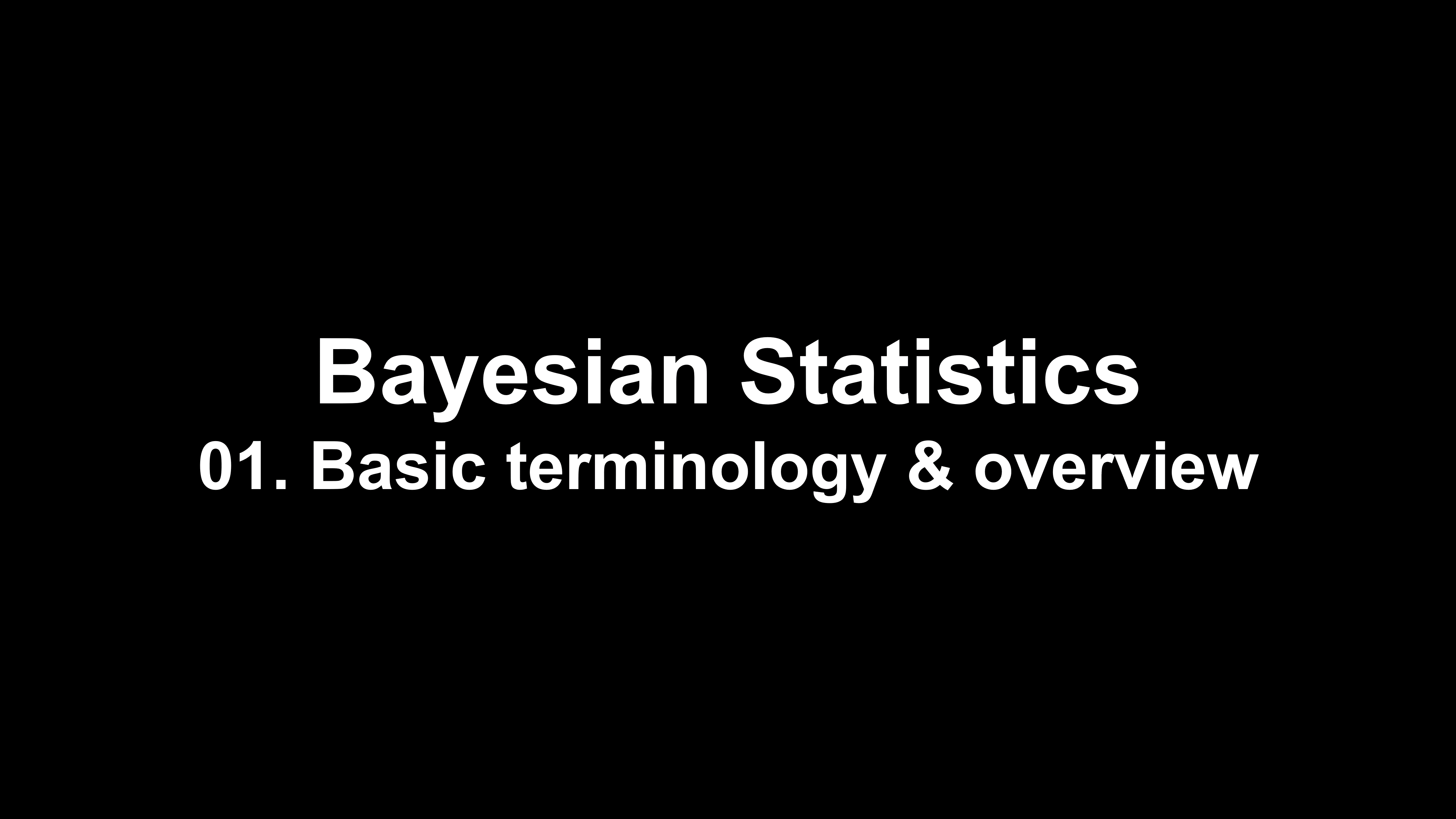### 1. Structure of generic bayesian inference procedure

• Design a proper prior $p(\theta)$: prior design is important for scientific applications, and various types of prior is used in different cases
• Non-informative prior, Spike-and-slab prior, ...
• Likelihood term $L(X|\theta)$, i.e. data generating mechanism takes account into contribution of dataset(observations)
• Posterior $\pi(\theta|X) \propto L(X|\theta)p(\theta)$ can be obtained in a closed form for some prior designs(conjugate priors), while most of the case we only can calculate 'kernel' of the distribution since $\pi(\theta|X) = \frac{L(X|\theta)p(\theta)}{\int L(X|\theta)p(\theta)d\theta}$ , and integral of the nominator is usually hard to calculate.
• Perform some statistical inferences on posterior distribution, for example calculate the posterior mean or credible interval.

### 2. Bayesian inference on posterior distribution

• Posterior mean: $E_{\pi}[\theta] = \int\pi(\theta|X)\theta d \theta \approx \sum_{\theta=1}^N{1 \over N}\pi(\theta|X)\theta$ (monte carlo estimate)
• Bayesian credible interval: $1-\alpha$ credible interval (a,b) for a,b, s.t. $\int_{a}^b\pi(\theta|X)d \theta=1-\alpha$
• HPD interval(highest posterior density interval) and its interpretation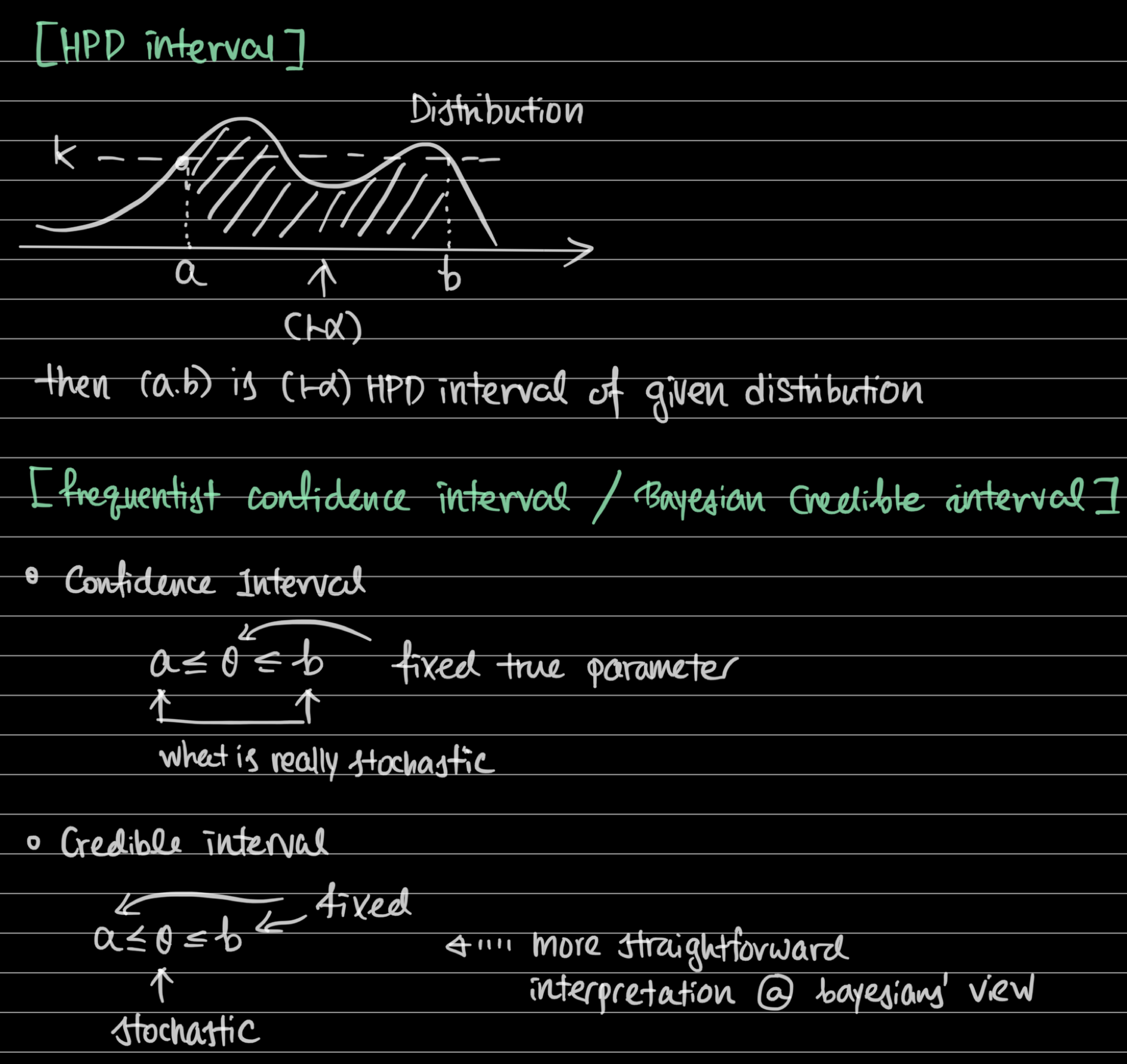### 3. Choosing priors

• If data size n increases, posterior becomes close to likelihood
• For large n, MLE ~ posterior mode(mean)
• Thus point estimate and uncertainty becomes close in bayesian & frequentist's view.
• But not always n is sufficiently large & similarity assumption requires some regularity conditions to be met.
• Prior does matter, and cause different result compared with MLE approach in bayesian inference.
• Several ways to choose priors
• Non-informative priors (e.g. Uniform distribution) : Same density across different parameter space
• When we don't have any pre-knowledge
• Bayesian inference & MLE will be similar
• Posterior mode = Maximum likelihood estimate when prior is non-informative
• Convenient priors(i.e. conjugate priors) : Analytically closed-form posteriors are preferred
• By choosing proper priors we can make posterior in a form of beta, normal, inverse-gamma distributions.
• Easy to calculate mean & credible interval
• Select conjugate priors
• Expert opinion : Realistic range of parameters exists(e.g. decay rate $\theta \in [0,1]$), pre-knowledge can be adopted
• Rule-based priors(e.g. Jeffrey's prior)

### 4. Conjugate priors

• Not always posterior is tractable in closed form, but special pairs of (prior, likelihood) can lead to tractable posterior
• Pairs of conjugate prior and likelihood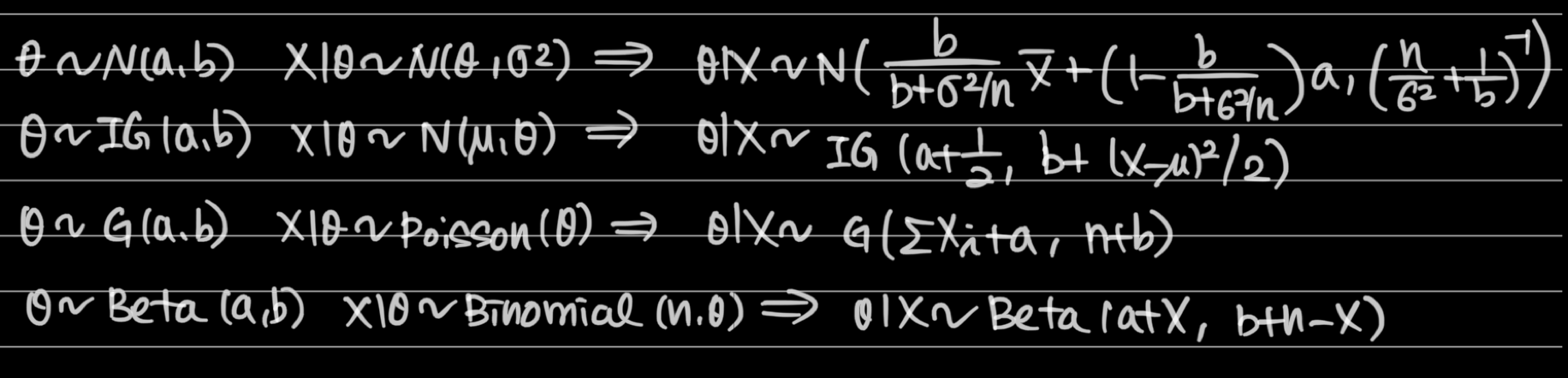• BE CAREFUL!
• IG(a,b): shape and rate parameter
• G(a,b): shape and rate parameter
• Mean of G(a,b) = ab: for shape and scale parameter
• Mean of IG(a,b) = b/(a-1): for shape and scale parameter

### 5. Jeffrey prior

• Prior which is invariant to transformations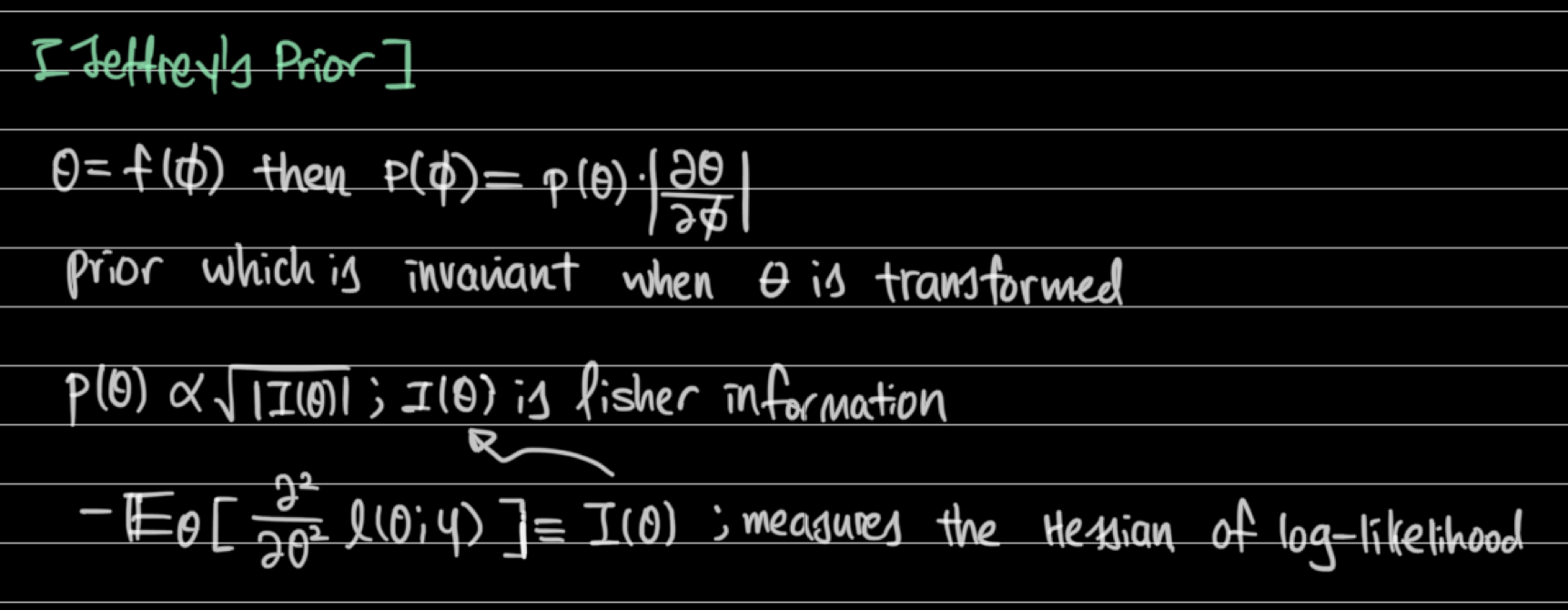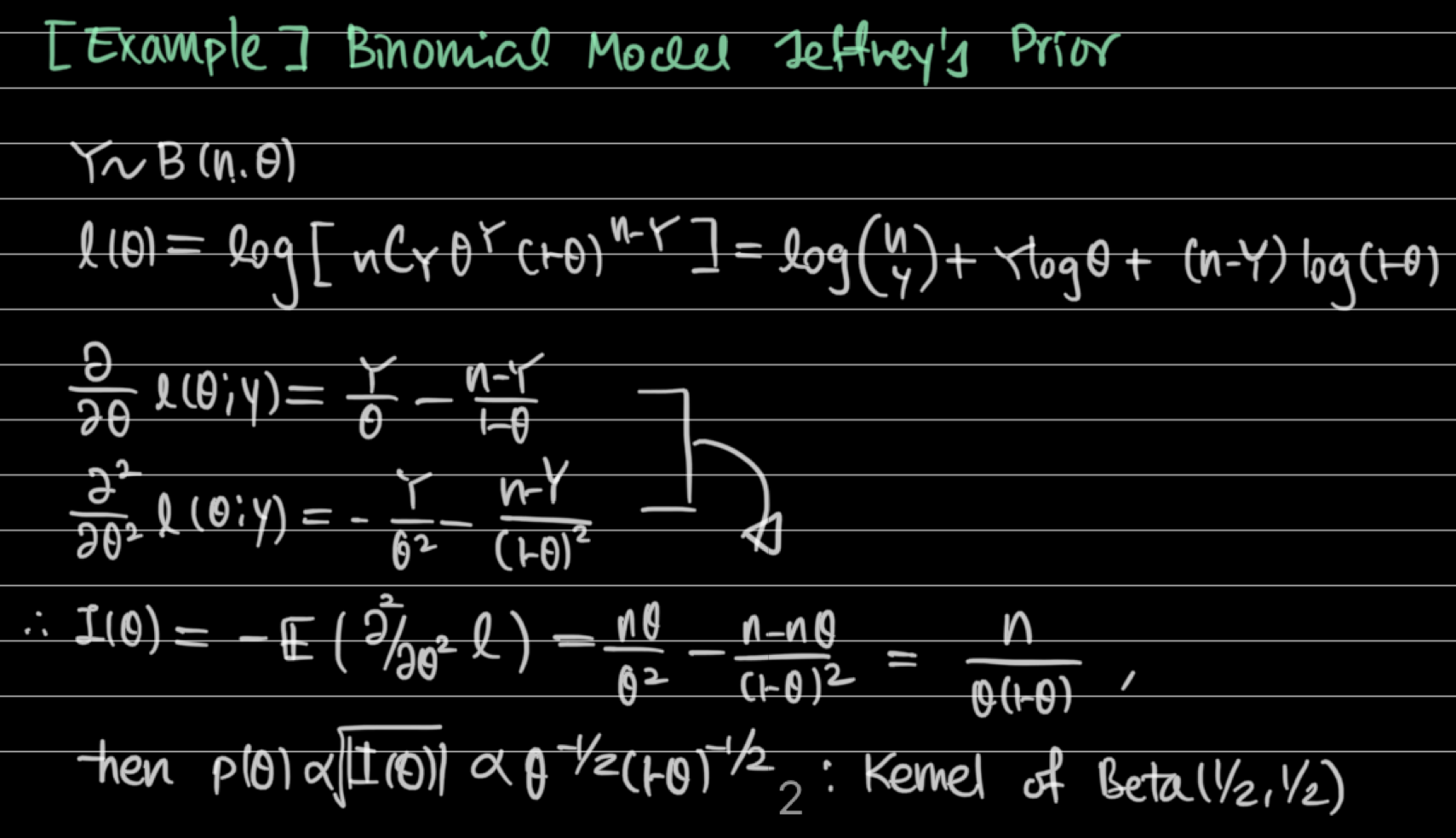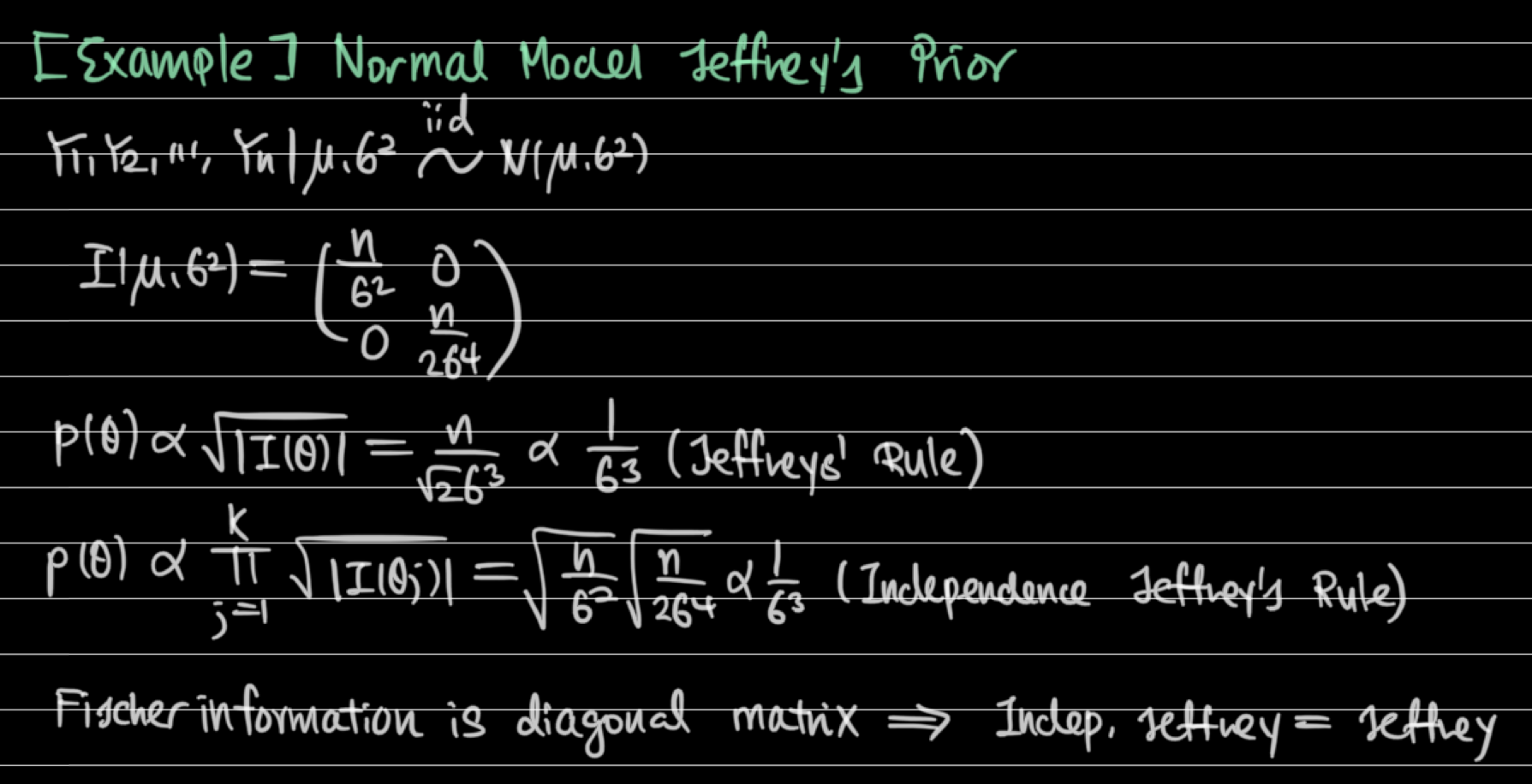### 5. Non-conjugate families

• Gibbs sampler
• Draw samples from full conditional of posterior distribution
• Metropolis-hastings algorithm
• Draw samples even if we don't know the full conditional of posterior distribution